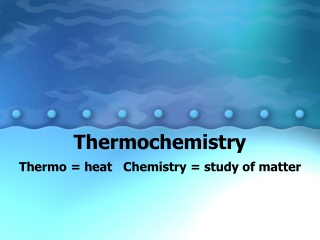DownloadDownload PresentationThermochemistry

# Thermochemistry

Download Presentation## Thermochemistry

- - - - - - - - - - - - - - - - - - - - - - - - - - - E N D - - - - - - - - - - - - - - - - - - - - - - - - - - -
##### Presentation Transcript

1. Thermochemistry Thermo = heat Chemistry = study of matter

2. Thermochemistry • Study of heat changes and energy that accompany chemical reactions and phase changes

3. Review of Energy • Capacity to do work or to create heat and or generate electricity • Types: • Chemical • Nuclear • Thermal • Radiant (light) • Electrical • Mechanical

4. Law of conservation of Energy • Energy can be converted from one form to another but it cannot be created or destroyed

5. Forms of energy: • Potential • Stored energy • Kinetic • Energy of motion

6. Chemical potential energy • Energy stored within the structural units of chemical compounds

7. Heat is energy (Q) • flows from an area of high heat to an area of low heat • Can be measured in calories, Calories, or Joules • 1000 cal = 1 Cal = 4.184 J

8. Heat energy (Q) • System: part of the universe being studied • Surroundings: everything outside of the system • Universe: system + surroundings

9. Heat energy (Q) Endothermic: • feels cold to touch • temperature decreases • heat absorbed by system • Q = +

10. Heat energy (Q) Exothermic: • feels warm to touch • temperature increases • heat released by system • Q = -

11. Specific heat capacity • C or Cp • The amount of energy required to raise the temperature of 1 gram of a substance 1ºC • Water= 4.18 J/g 0C • Glass=.50 J/g 0C

12. Specific heat capacity • Measure of a substance’s resistance to temperature change • High=resistant (water) • Low=easy (metals, glass, etc.)

13. Equation Q = m C ∆T - Qis the heat absorbed or released in Joules -m is mass in grams -C is the specific heat in J/g/ºC -∆T is the temperature change in ºC

14. (Q) = m C (DT) Temperature change (final temp minus initial temp) Specific heat capacity in J/g-oC mass

15. When 1982 g of water underwent a temperature change from 23.677oC to 27.482oC, how much energy in kJ did the water absorb? The specific heat of water is 4.184 J/(g-oC). Show all work!

16. How much energy in J is required to raise the temperature of 500.0 g of copper from 22.8oC to 100.0oC? The specific heat of copper is 0.387 J/g/oC. Show all work!

17. Measuring heat exchange Calorimeter- insulated device used for measuring the amount of heat absorbed or released during a reaction

18. Calorimeter

19. Calorimeter In a closed system: Heat loss = Heat gain Q lost = Q gained m C∆ T = m C ∆ T

20. Enthalpy • (∆H) heat content of a system at constant pressure • Can measure changes in enthalpy during a reaction

21. Enthalpy heat of reaction∆ Hrxn ∆ Hrxn = Hfinal - Hinitial Or ∆ Hrxn = Hproducts - Hreactants

22. Thermochemical Equations • Used to calculate and express heat changes. • The physical states of the reactants and products in this equation is important! • Example: CH4(g) + 2O2(g)→ CO2(g) + 2H2O(l) ∆H = -890.2 kJ

23. Exothermic rxn: ∆ Hrxn is < 0 ∆ Hrxn is negative

24. Endothermic rxn: ∆ Hrxn is > 0 ∆ Hrxn is positive